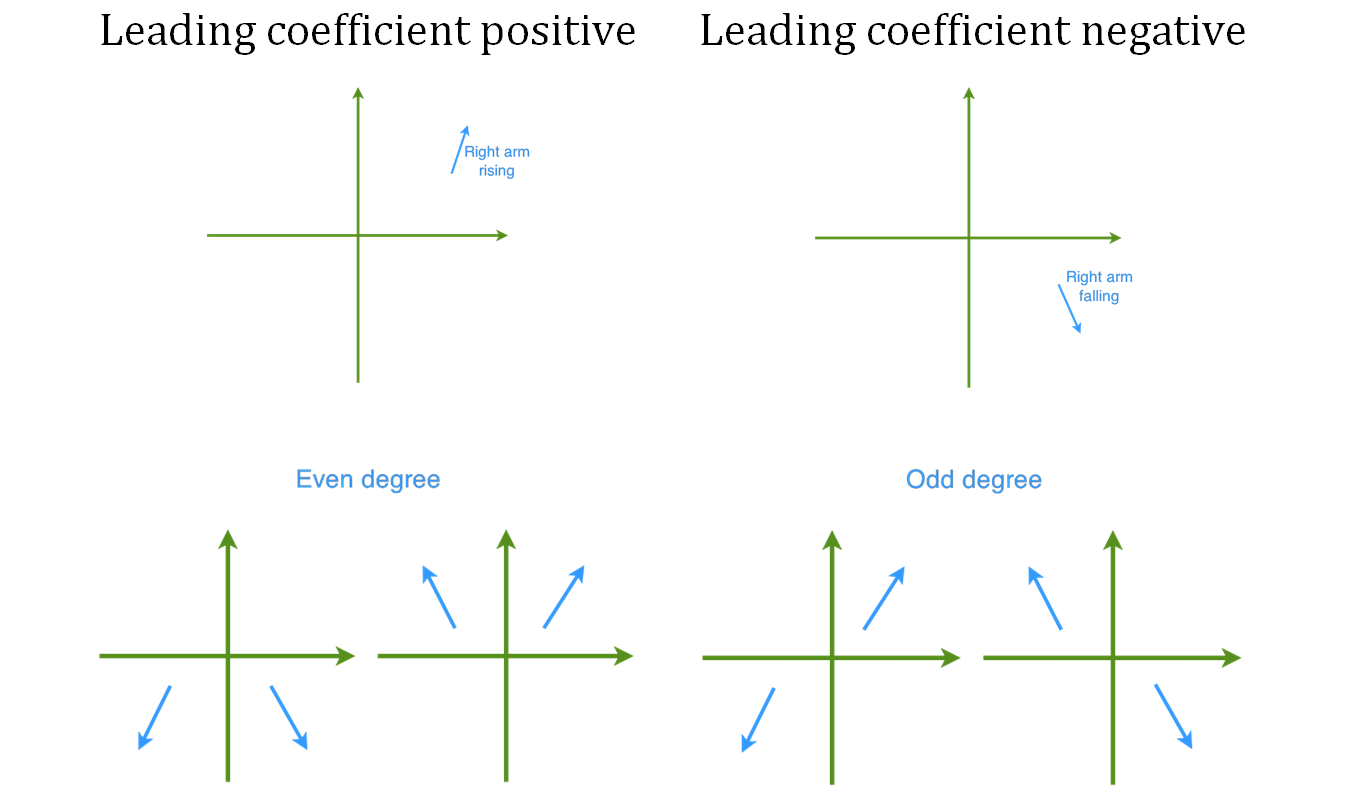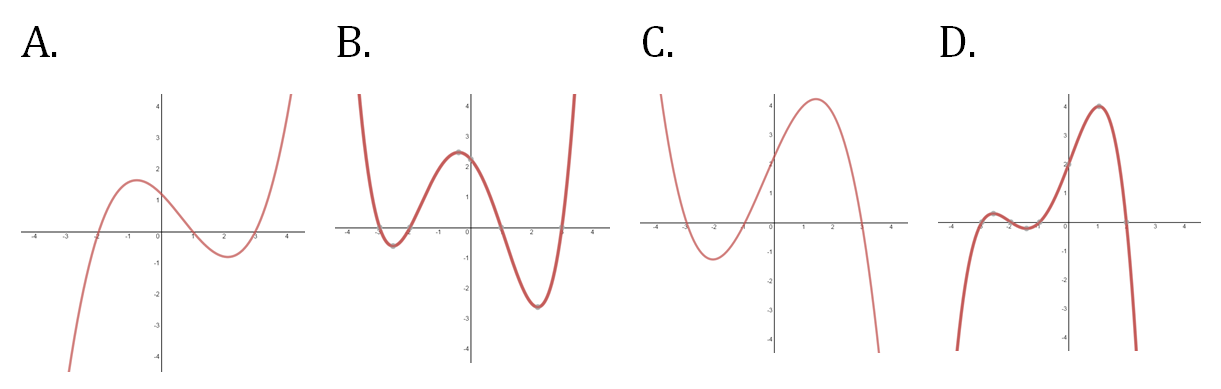# Characteristics of polynomial graphs

### Characteristics of polynomial graphs

#### Lessons• 1.
Investigating the Graphs of Polynomial Functions
Recognize the characteristics of polynomial graphs from the following quadratic and cubic functions:
i)
$P\left( x \right) = {x^2} - 3x - 10$
ii)
$P\left( x \right) = - {x^2} + 3x + 10$
iii)
$P\left( x \right) = {x^3} - 3{x^2} - 10x$
iv)
$P\left( x \right) = - {x^3} + 3{x^2} + 10x$

• 2.
Graphing Polynomials
Sketch the following polynomials:
a)
$P(x)=(x-3)(x-1)(x+2)(x+5)$

b)
$P(x)=-(x-5)(x-2)(x+1)(x+4)(x+6)$

c)
$P(x)=-x^2-2x+8$

d)
$P(x)=x^3-8x^2+15x$

• 3.
Identifying Polynomial Graphs
Which of the following could be the graph of a quadratic function with a negative leading coefficient?